# GSEB Solutions Class 10 Maths Chapter 6 Triangle Ex 6.5

Gujarat Board GSEB Solutions Class 10 Maths Chapter 6 Triangle Ex 6.5 Textbook Questions and Answers.

## Gujarat Board Textbook Solutions Class 10 Maths Chapter 6 Triangle Ex 6.5

Question 1.
Sides of triangles are given below. Determine which of these are right triangle. Write the length of its hypotenuse.
(i) 7 cm, 24 cm, 25 cm
(ii) 3 cm, 8 cm, 6 cm
(iii) 50 cm, 80 cm, 100 cm
(iv) 13 cm, 12 cm, 5 cm
Solution:
(i) 7 cm, 24 cm, 25 cm.
Here 72 = 49cm
242 = 576 cm
252 = 625 cm
we observed that
49 + 576 = 625
72 + 242 = 252
The given triangle is right angled triangle.
Hypotenuse = 25 cm

(ii) 3 cm, 8 cm, 6 cm
Here 32 = 9cm
82 = 64 cm
62 = 36 cm
we observed that
32 + 62 + 82
The given triangle is not right angled triangle.

(iii) 50 cm, 80 cm, 100 cm.
Here 502 = 2500 cm
802 = 6400 cm
1002 = 10,000 cm
We observed that
502 + 802 ≠ 1002
The given triangle is not right angled.

(iv) 13 cm, 12 cm, 5 cm.
Here 132 = 169 cm
122 = 144 cm
52 = 25 cm
we observed that
122 + 52 = 132
∴ The given triangle is not right angled.
Hypotenuse = 13 cmQuestion 2.
PQR is a triangle right angled at P and M is a point on QR such that PM⊥QR. Show that
PM2 = QM.MR.
Solution: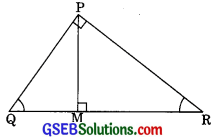In ∆QMP and ∆QPR
∠QMP = ∠QPR (each 90°)
∠PQM = ∠PQR (Common)
∴ ∆PQM – ∆QPR ………(1) (by AA similarity)

Again in ∆PMR and ∆QPR
∠PMR = ∠QPR (each 900)
∠PRM = ∠QRP (Common)
∴ ∆PMR – ∆DQPR ………(2)
From (1) and (2)
∆QMP ~ ∆PMR
$$\frac{Q M}{P M}=\frac{P M}{R M}$$
(The corresponding sides of similar triangles are proportional)
PM2 = QM.RM

Question 3.
In the figure ABD is a triangle, right angled at A and AC ⊥BD. Show that
(i) AB2 = BC BD
(ii) AC2 = BC DC
(iii) AD2 = BD . CD (CBSE 2010)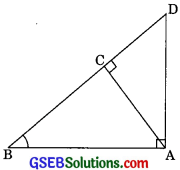Solution:
∠ABC = ∠ABD (common)
∆BAC ~ ∆BDA (AA similarity)
$$\frac{A B}{D B}=\frac{B C}{B A}$$
(Corresponding sides are proportional)
⇒ AB2 = BC.BD(ii) In ∆ABC
∠BAC + ∠CBA = 90° ………(1) (by angle sum property)
In ∆ABD
∠BDA + ∠CBA = 90° …….(2)
From eqn (1) and (2)
∠BAC + ∠CBA = ∠BDA + ∠CBA
∠BAC = ∠BDA
In ∆ACB and ∆DCA
∠ACB = ∠DCA (each 900)
∠BAC = ∠BDA
(proved above from eqn (3))
∴ ∆ACB ~ ∆DCA (by AA similarity)
$$\frac{AC}{DC}=\frac{BC}{AC}$$
(corresponding sides of two similar triangle are proportional)
⇒ AC2 = BC.DC

∠DAB = ∠DCA (each 900)
∴ ∆ADB ~ ∆CDA (by AA similarity)
Hence = $$\frac{AD}{CD}=\frac{BD}{CD}$$
(Corresponding sides of two similar triangles are proportional)Question 4.
ABC is an isosceles triangle right angled at C. Prove that AB2 = 2AC2. (CBSE 2012)
Solution:
We have ∆ABC is right angled such that
∠C = 90° and AC = AB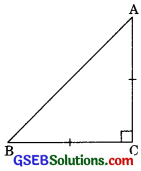Applying Pythagoras theorem
– AC2 + BC2
= AC2 + AC2 (BC = AC given)
Thus AB2 = 2AC2

Question 5.
ABC is an isosceles triangle with AC = BC, If BC2 = 2AC2, prove that ABC is a right triangle.
Solution:
We have ∆ABC such that AB = ACAlso AB2 = 2AC2 = AC2 + AC2
AB2 = BC2 +AC2(BC =AC given)
∠ACB = 90° (by converse of Pythagoras theorem)
i.e. ∆ABC is a right angle triangle

Question 6.
ABC is an equilateral triangle of side 2a. Find each of its attitudes.
Solution:
It is given that LABC is an equilateral triangle in whichAB = AC = CB = 2a
Let us draw AD ⊥ BC
Now in ∆ABD and ∆ACD
AB = AC (Side of equilateral triangle)
∴ ∆ABD ≅ ∆ACD (by RHS)
∴ BD = CD (by CPCT)
i.e. D is a mid point of BC
Hence BD = $$\frac {2a}{2}$$ = a
Now in right ∆ABD
AD = $$\sqrt { 3 }$$
Similarly BE = CF = $$\sqrt { 3 }$$Question 7.
Prove that the sum of the squares of the sides of a rhombus is equal to the sum of the squares of its diagonals. (CBSE 2012) of its diagonals. (CBSE 2012)
Solution:
Given: ABCD is a rhombus i.e. AB = BC = CD =
DA whose diagonals AC and BD intersect at O.
To prove: AC2 + BD2
= AB2 + BC2 + CD2 + DA2Proof: We know that diagonals of a rhombus bisect each other at right angles.
∴ OA = OC and OB = OD
Now in right ∆AOB
AB2 = OA2 + OB2 (by Pythagoras theorem)
In right ∆BOC
BC2 = OB2 + OC2……..(2)
Similarly in ∆COD
CD2 = OC2 + OD2
and in right ∆AOD
Now adding eqn (1), (2), (3) and (4) we get
AB2 + BC2 + CD2 + DA2
= OA2 + OB2 + OC2 + OB2 + OD2 + OC2 + OD2 + OA2
= 20A2 + 20B2 + 20C2 + 20D2
= 20A2 + 20B2 + 20A2 + 20B2
= 40A2 + 40B2
= (20A)2 + (20B)2 + AB2 + BC2 + CD2 + DA2
= AC2 + BD2
(20A = AC and 20B = BD)Question 8.
In the figure, O is a point in the interior of a triangle ABC, OD ⊥ BC, OE ⊥ AC and OF ⊥ AB show that
(i) OA2 + OB2 + OC2 – OD2 – DE2 – OF2 = AF2 + BD2 + CE2
(ii) AF2 + BD2 + CE2 = AE2 + CD2 + BF2Solution:
In the given figure, 0 is in the interior of ∆ABC,
OD ⊥ BC, OF ⊥AB, OE ⊥AC
To prove:
(i) OA2 + OB2 + OC2 + 0D2 + 0E2 + OF2 = AF2 + BD2 + CE2
(ii) AF2 + BD2 + CE2 = 2 + CD2 + BF2
Construction: Join OA, OB and OC
Proof: In right ∆AOF
OA2 = AF2 + OF2 (by Pythagoras theorem)
AF2 = OA2 + OF2 ……….(1)

In right ∆OBD
OB2 + BD2 + OD2
BD2 = OB2 + OD2 ………..(2)

In right ∆OCE
OC2 = CE2 + OE2
CE2 = OC2 + OE2
Adding eqn (1), (2) and (3) we get
AF2 + BD2 + CE2 = OA2 + OB2 + OC2 + OD2 + OE2 – OF2 ………..(3)(ii) In right ∆OBD
OB2 = OD2 + BD2
(by Pythagoras theorem)
⇒ BD2 = OB2 – OD2 ……….(1)

In right triangle ∆OCD
OC2 = OD2 + CD2
(by Pythagoras theorem)
⇒ CD2 = OC2 – OD2 ……….(2)

Subtracting eqn (2) from eqn (1)
BD2 – CD2 = OB2 – OD2 – OC2 – OD2
BD2 – CD2 = OB2 – OC2 ……….(3)
Similarly in right triangle OCE and OAE we get
CE2 = OC2 – OE2 ………(4)
(by Pythagoras theorem)
AE2 = OA2 – OE2 ………(5)

Subtracting eqn (5) from eqn (4)
CE2 – AE2 = OC2 – OE2 – OA2 + OE2
⇒ CE2 – AE2 = OC2 – OA2 ……….(6)

And in right triangle OAF and OBF
AF2 = OA2 – OF2 ……….(7)
BF2 = OB2 – OF2 ………..(8)

Subtracting eqn (8) form (7)
AF2 – BF2 = AO2 – OF2 – OB2 + OF2
2 – BF2 = OA2 – OB2 ………..(9)Adding eqn (3), (6) and eqn (9) we get
BD2 + CE2 + AF2 – CD2 – AE2 – BF2
⇒ OB2 + OC2 + OA2 – OC2 – OA2 – 0B2
BD2 + CE2 + AF2 – CD2 – AE2 – BF2 = O
BD2 + CE2 + AF2 = CD2 + AE2 + BF2

Question 9.
A ladder 10 m long reaches a window 8 m above the ground. Find the distance of the foot of the ladder from base of the wall.
Solution:
Let AB is a ladder and A is the foot of the ladder BC is the wall.Now in right triangle ABC
AB2 = AC2 + BC2
(by Pythagoras theorem)
(10)2 + AC2 + 82
⇒ 100 – 64 = AC2
⇒ AC2 = 36
⇒ AC = 6m
Hence, the distance of the foot of the ladder from the base of wall is 6 m.Question 10.
A guy wire attached to a vertical pole of height 18 m is 24 m long and has a state attached to the other end. How far from the base of the pole should the stake be driven so that the wire will be taut.
Solution:
Let AB is the wire and BC is the vertical pole. The point A is the stake.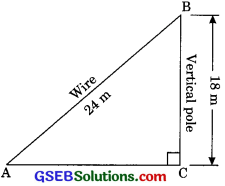Now in right triangle ABC
AB2 = AC2 + BC2
242 = AC2 + 182
AC2 = 242 – 182
AC2 = (24 – 18)(24 + 18)
AC2 = 6 x 42
AC = $$\sqrt{6 \times 42}$$
AC = $$\sqrt{6 \times 6 \times 7}$$
⇒ AC = 6$$\sqrt{6 \times 42}$$m.Question 11.
An aeroplane leaves an airport and flies due north at a speed of 1000 km per hour. At the same time another aeroplane leaves the same airport and flies due west at a speed of 1200 km per hour. How far apart will be the two plane after 1 $$\frac{1}{2}$$ hours?
Solution:
Let the point A represent the airport. First plane flies due North with speed of 1000 km/h.
Distance of plane after 1$$\frac{1}{2}$$ hour from airport = speed x time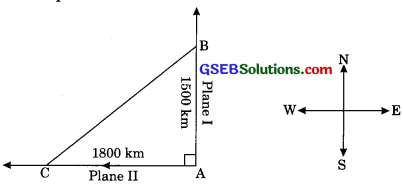Distance due North = 1000 x 1$$\frac{1}{2}$$
= 1000 x $$\frac{3}{2}$$
= 1500 km
Similarly, distance of plane after 11$$\frac{1}{2}$$ hour From airport due west
= 1200 x 11$$\frac{1}{2}$$ = 1200 x $$\frac{3}{2}$$
= 1800 km
Hence in right triangle ABC
BC2 = AB2 + AC2
BC2 = (1500)2 + (1800)2
BC2 = 22500 + 32400
BC2 = 54900
BC = $$\sqrt{54900}$$ km
BC = $$\sqrt{61 x 90000}$$
BC = 300 $$\sqrt{61}$$m.Question 12.
Two poles of height 6 m and 11 m stand on a plane ground. If the distance between the feet of the poles is 12 m, find the distance between their tops.
Solution:
Let AB and CD be the two poles. Distance between their feet is 12 m. Height of pole 1st
AB = 11 m and height of pole lind CD = 6 m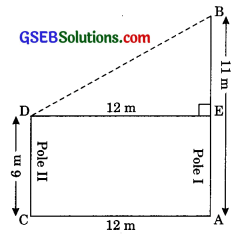AB = 11m
CD = 6m
BB = AB – AE (AE = CD)
⇒BE =11 – 6
⇒BE = 5m

In ∆BED
BD2 = DE2 + BE2
= 122 + 52 = 144 + 25
⇒ BD2 = 169
⇒ BD = 13m
Thus the distance between tops is 13 metres.

Question 13.
D and E are points on the sides CA and CB respectively of a triangle ABC, right angled at C. Prove that AE2 + BD2 = AB2 + DE2
Solution:
Given: ∆ABC in which ∠C = 90°, D and E are the mid prints on the sides AC and BC.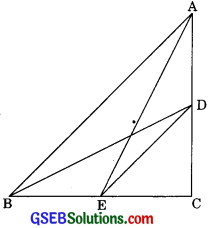To prove: AB2 + BD2 = AB2 + DE2
Proof: In right angle AACB
AB2 = AC2 + BC2 (by Pythagoras theorem)
In DCE
DE2 = CD2 + CE2 ………(2)
(by Pythagoras theorem)
We get AB2 + DE2 = AC2 + BC2 + CD2 + CE2
AB2 + DE2 = (AC2 + CE2) +(BC2 + CD2) …….(3)

Now in ∆ACE
AE2 = AC2 + CE2 ……. (4)
In ∆BCD
BC2 + CD2 = BD2 ……(5)
from eqn (3), (4) and (5)
AB2 + DE2 = AE2 + BD2

Question 14.
The perpendicular from A on side BC at D of a ¿ABC intersects BC at D such that DB = 3CD (see figure). Prove that 2AB2 = 2AC2 + BC2.
Solution:
Given: ∆ABC such that AD ⊥ BC. The position of D is such that BD = 3 CD.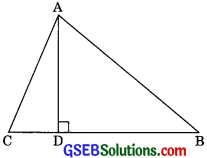In right ∆ABD, we have
AB2 = AD2 + BD2 …….(1) (by Pythagoras theorem)
Similarly from right IACD,
AC2 = AD2 + CD2 ……(2)
Note that, BC = DB + CD
BC = 3CD + CD (BD = CD given)
BC = 4 CD
Subtracting eqn (2) from eqn (1), we get
⇒ AB2 – AC2 = BD2 – CD2
⇒ AB2 – AC2 = (BD + CD) (BD – CD)
⇒ AB2 – AC2 = BC (3CD – CD)
⇒ AB2 – AC2 = BC x 2CD
⇒ AB2 – AC2 = BC x $$\frac{2 \times \mathrm{BC}}{4}{r}$$ CD = $$\frac{BC}{4}$$
⇒ AB2 – AC2 = $$\frac{\mathrm{BC} \times \mathrm{BC}}{2}$$
⇒ 2AB2 – 2AC2 = BC2
⇒ AB2 = 2AC2 + BC2

Question 15.
In an equilateral triangle ABC, D is a point on side BC such that BD = BC. Prove that
Solution:
Given: AABC is an equilateral triangle.BD = $$\frac{1}{3}$$ BC
Construction: Draw AP⊥BC
Proof: In right AAPB, we have
AB2 =AP2 + BP2 …….(1) (by Pythagoras theorem))
Now, in right triangle APD, we have
(by Pythagoras theorem)
From eqn (1) and (2) we get
AB2 =AD2 – DP2 + BP2
AB2 =AD2 – DP2 + (BP = BC)
AD2 = AB2 + DP2 – 4
Note that, DP = BP – BD
DP = $$\frac{1}{2}$$BC – $$\frac{1}{3}$$BC = $$\frac{1}{6}$$BC in (3),gives
Substituting value of DP = $$\frac{1}{6}$$BC
AD2 = AB2 + ($$\frac{1}{6}$$BC2)2 – $$\frac{\mathrm{BC}^{2}}{4}$$
⇒ AD2 = AB2 + $$\frac{\mathrm{BC}^{2}}{36}$$ – $$\frac{\mathrm{BC}^{2}}{4}$$
⇒ AD2 = AB2 + $$\frac{\mathrm{BC}^{2}-9 \mathrm{BC}^{2}}{36}$$
⇒ AD2 = AB2 – $$\frac{8 \mathrm{BC}^{2}}{36}$$
⇒ AD2 = AB2 – $$\frac{2 \mathrm{BC}^{2}}{9}$$
⇒ AD2 = AB2 – $$\frac{2 \mathrm{2AB}^{2}}{9}$$ (BC = AB = AC)
⇒ 9AD2 = 9AB2 – 2AB2

Question 16.
In an equilateral triangle, prove that three times the square of one side is equal to four times the square of one of its altitude.
Solution:
Given: ABC is an equilateral triangle,BD = DC = $$\frac{BC}{2}$$ ………(1) (D is the midpoint)
AB2 = AD2 + $$\left(\frac{\mathrm{BC}}{2}\right)^{2}$$[(Using (1)]
AB2 = AD2 + $$\frac{\mathrm{BC}^{2}}{4}$$ (∆ABC is an equilateral)
= AB2 = AD2 + $$\frac{\mathrm{AB}^{2}}{4}$$ (asAB = BC)

Question 17.
Tick the correct answer and justify: In AB = 6$$\sqrt {3}$$ cm, AC = 12 cm, and BC = 6 cm. The angle B is
(a) 120°
(b) 60°
(c) 90°
(d) 45°
Solution:
Wehave AB = 6$$\sqrt {3}$$ cm,AC = 12cm, and BC = 6 cm
AB2 = (6$$\sqrt {3}$$)2 = 36 x 3
AB2 = 108
AC2 = 122 = 144
BC2 = 2 = 36
Since 144 = 108 + 36
i.e. AC2 = AB2 + BC2
Hence the given triangle is right angle triangle at B
∠B = 90°
∴ The correct answer is (c).